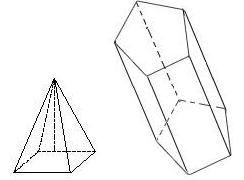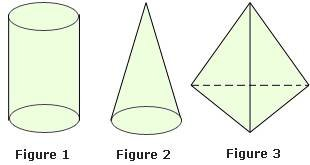## Definition Of Three Dimensional Figures

Three-dimensional figures are those figures that do not lie in a plane

The dimensions of three-dimensional figures are length, width, and height

### Examples of Three Dimensional Figures### Solved Example on Three-dimensional Figures

#### Ques: Which of the three-dimensional figures do not have a polygonal base?##### Choices:

A. Figure 1
B. Figure 2
C. Figure 3
D. Figure 1 & Figure 2